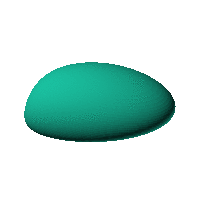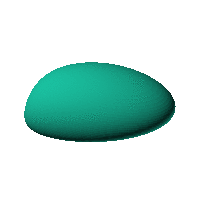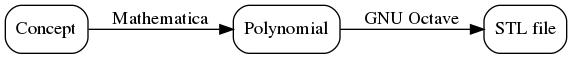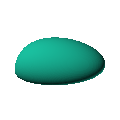Blob Farm# blob67The blob in all its glory:This is aimed at being a case that has an obvious way of sitting on a surface and a recessed area for a hole for a USB or other cable. The formula was generated in Mathematica but GNU Octave was used to generate an STL file from the formula.Click on the snapshot to download the blob's stl file.Octave Code:
```  # name of the blob
project = "blob67";

# function at origin must be <0, and >0 far enough away.  w=0 defines the surface
function w = f(x2,y2,z2,c,r)  # three lobed toroid with low profile bumps and flat top
x = (x2-c(1))/r(1);
y = (y2-c(2))/r(2);
z = (z2-c(3))/r(3);

w = 3.5*(7.14286.*x.^2+(-4.28571).*x.^3+x.^4+( ...
-4.28571).*x.^2.*z+7.14286.*z.^2+(-17.1429).*x.*z.^2+ ...
8.0.*x.^2.*z.^2+(-17.1429).*z.^3+16.0.*z.^4+2.03175.* ...
y.^2+(-17.1429).*x.*y.^2+8.*x.^2.*y.^2+(-17.1429).*z.* ...
y.^2+32.0.*z.^2.*y.^2+16.0.*y.^4);

endfunction;

step = 2;  # grid pitch in mm  start with 4mm to see the shape quickly.  Once you have it just right, change to 2mm for printing

c_outer = [-10,0,-40]; # for moving center (origin) of blob
r_outer = [70,70,70];# for scaling blob

xmin = 0 ;  # avoid the origin because the inverse of the gradient is NaN there
xmax = 250 ;

source("../octave/func2stl_v01.m");  # do all the calculations
```
GNU Octave